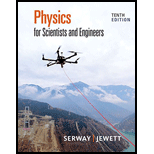Chapter 25, Problem 6PPhysics for Scientists and Enginee...

10th Edition
Raymond A. Serway + 1 other
ISBN: 9781337553278

Solutions

Chapter
SectionPhysics for Scientists and Enginee...

10th Edition
Raymond A. Serway + 1 other
ISBN: 9781337553278
Textbook Problem

Review. A small object of mass m carries a charge q and is suspended by a thread between the vertical plates of a parallel-plate capacitor. The plate separation is d. If the thread makes an angle θ with the vertical, what is the potential difference between the plates?

To determine
The potential difference between the plates.

Explanation

Given information: Mass of small object is m , charge stored by the capacitor is q , distance separated by the plate is d , angle suspended by a thread is θ .

Explanation:

The free body diagram is shown below:

Figure (1)

Write the expression for the applied force.

E=FqF=Eq

Here,

F is the applied force.

E is the applied electric field.

q is the charge stored by the capacitor.

Write the expression for the weight of the small object.

W=mg

Here,

W is the weight of the small object.

m is the mass of small object.

g is the acceleration due to gravity.

Apply Newton’s law in vertical direction,

W=Tcosθ (1)

Here,

T is the tension acting on the thread.

θ is the angle suspended by a thread.

Substitute mg for W in equation (1) to find T ,

mg=TcosθT=mgcosθ (2)

Apply Newton’s law in horizontal direction,

F=Tsinθ (3)

Substitute Eq

Still sussing out bartleby?

Check out a sample textbook solution.

See a sample solution

The Solution to Your Study Problems

Bartleby provides explanations to thousands of textbook problems written by our experts, many with advanced degrees!

Get Started

Methylmercuryis a toxic industrial pollutant that is easily destroyed by cooking. T F

Nutrition: Concepts and Controversies - Standalone book (MindTap Course List)

Explain why somebut not allof an organism's genes are expressed.

Biology: The Unity and Diversity of Life (MindTap Course List)

Technically, there are no salts in seawater. How can that be?

Oceanography: An Invitation To Marine Science, Loose-leaf Versin